Function Repository Resource:

Recover a Jacobi matrix from a list of abscissa-weight pairs

Contributed by: Jan Mangaldan
 ResourceFunction["QuadratureWeightsToJacobiMatrix"][{{x1,w1},{x2,w2},…}] returns a list {j,c}, where j is the Jacobi matrix corresponding to the quadrature rule represented by the abscissa-weight pairs {xi,wi} and normalization factor c.

## Examples

### Basic Examples (2)

The resource function GaussianQuadratureWeights gives a list of abscissa-weight pairs for Gauss-Legendre quadrature:

 In:=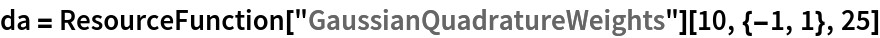Out=Recover the corresponding Jacobi matrix and normalization factor:

 In:=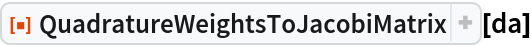Out=### Scope (4)

 In:=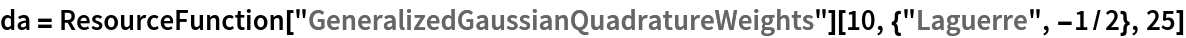Out=Recover the Jacobi matrix and normalization factor from the quadrature data:

 In:=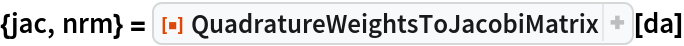Out=Compare the recovered Jacobi matrix with the result of the resource function JacobiMatrix:

 In:=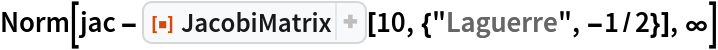Out=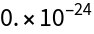The normalization factor is equal to the sum of the weights:

 In:=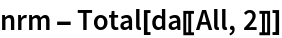Out=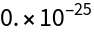### Applications (2)

QuadratureWeightsToJacobiMatrix can be used even on non-Gaussian quadrature rules. Build a Jacobi matrix from a Newton-Cotes rule:

 In:=Out=The resulting Jacobi matrix is unsymmetric because some the weights of the quadrature rule are negative:

 In:=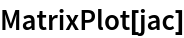Out=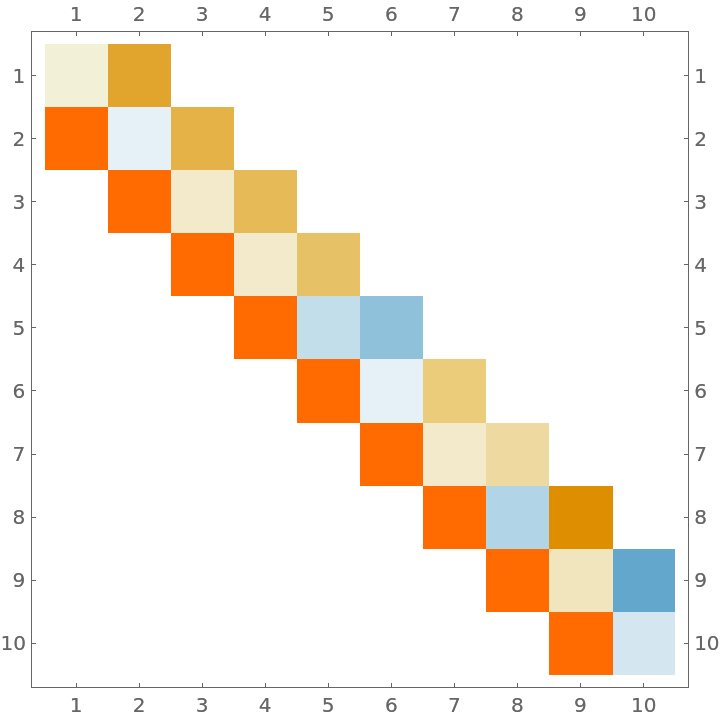A Clenshaw-Curtis rule whose weights are all positive will yield a symmetric Jacobi matrix:

 In:=Out=In:=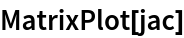Out=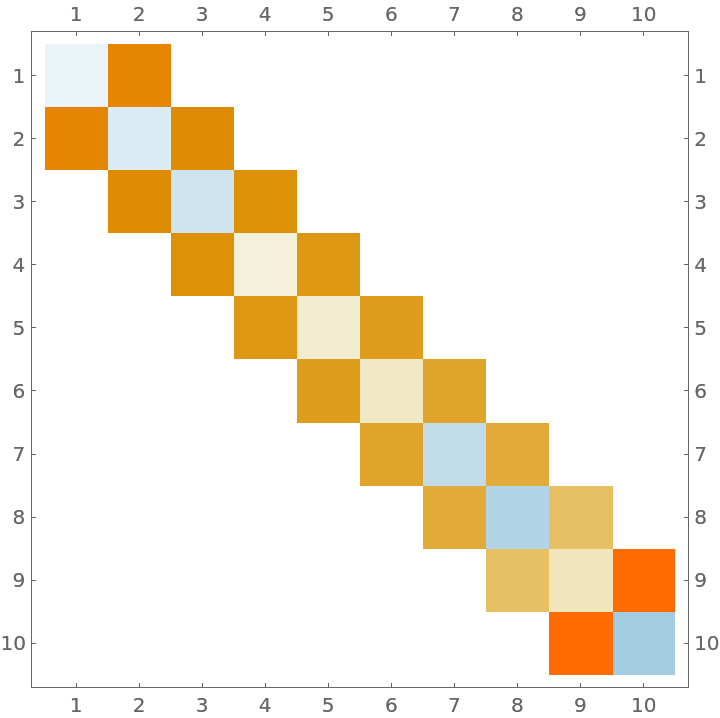## Version History

• 1.0.0 – 06 April 2021